JMSLTM Numerical Library 7.2.0
com.imsl.stat

## Class ARAutoUnivariate

• All Implemented Interfaces:
Serializable

```public class ARAutoUnivariate
extends Object
implements Serializable```
Automatically determines the best autoregressive time series model using Akaike's Information Criterion.

`ARAutoUnivariate` automatically selects the order of the AR model that best fits the data and then computes the AR coefficients. The algorithm used in `ARAutoUnivariate` is derived from the work of Akaike, H., et. al (1979) and Kitagawa and Akaike (1978). This code was adapted from the UNIMAR procedure published as part of the TIMSAC-78 Library.

The best fit AR model is determined by successively fitting AR models with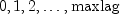autoregressive coefficients. For each model, Akaike's Information Criterion (AIC) is calculated based on the formula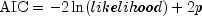Class `ARAutoUnivariate` uses the approximation to this formula developed by Ozaki and Oda (1979),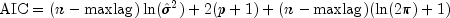where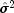is an estimate of the residual variance of the series, commonly known in time series analysis as the innovation variance and n is the number of observations in the time series `z`, n`=z.length`. By dropping the constant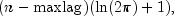the calculation is simplified to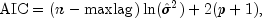The best fit model is the model with minimum AIC. If the number of parameters in this model selected by `ARAutoUnivariate` is equal to the highest order autoregressive model fitted, i.e., p=``` maxlag```, then a model with smaller AIC might exist for larger values of `maxlag`. In this case, increasing `maxlag` to explore AR models with additional autoregressive parameters might be warranted.

Method `setEstimationMethod` can be used to specify the method used to calculate the AR coeficients. If `setEstimationMethod` is set to `METHOD_OF_MOMENTS`, estimates of the autoregressive coefficients for the model with minimum AIC are calculated using method of moments as described in the `ARMA` class. If ```LEAST_SQUARES ``` is specified, the coefficients are determined by the method of least squares applied in the form described by Kitagawa and Akaike (1978). If `MAX_LIKELIHOOD` is specified, the coefficients are estimated using maximum likelihood as described in the `ARMAMaxLikelihood` class.

The Java Logging API can be used to trace the execution of ``` ARAutoUnivariate```. The name of this logger is ``` com.imsl.stat.ARAutoUnivariate```. Accumulated levels of detail correspond to Java's FINE, FINER, and FINEST logging levels with FINE yielding the smallest amount of information and FINEST yielding the most. The levels of output yield the following:

 Level Output FINE A message on entering and exiting method ``` compute```. FINER All of the messages in `FINE`, a message entering and exiting the `compute` method, plus the final computations. FINEST All of the messages in `FINER`, plus the intermediate computations.
Wolfer Sunspot Example, Wolfer Sunspot Example with Logging and Forecasting, Serialized Form
• ### Nested Class Summary

Nested Classes
Modifier and Type Class and Description
`static class ` `ARAutoUnivariate.TriangularMatrixSingularException`
The input triangular matrix is singular.
• ### Field Summary

Fields
Modifier and Type Field and Description
`static int` `LEAST_SQUARES`
Indicates that least-squares should be used for estimating the coefficients in the time series.
`static int` `MAX_LIKELIHOOD`
Indicates that maximum likelihood should be used for estimating the coefficients in the time series.
`static int` `METHOD_OF_MOMENTS`
Indicates the method of moments should be used for estimating the coefficients in the time series.
• ### Constructor Summary

Constructors
Constructor and Description
```ARAutoUnivariate(int maxlag, double[] z)```
`ARAutoUnivariate` constructor.
• ### Method Summary

Methods
Modifier and Type Method and Description
`void` `compute()`
Determines the autoregressive model with the minimum AIC by fitting autoregressive models from 0 to `maxlag` lags using the method of moments or an estimation method specified by the user through ``` setEstimationMethod```.
`double[][]` `forecast(int nForecast)`
Returns forecasts and associated confidence interval offsets.
`double` `getAIC()`
Returns the final estimate for Akaike's Information Criterion (AIC) at the optimum.
`double[]` `getAR()`
Returns the final auto regressive parameter estimates at the optimum AIC using the estimation method specified in ```setEstimationMethod ```.
`int` `getBackwardOrigin()`
Returns the maximum backward origin.
`double` `getConfidence()`
Returns the confidence level for calculating confidence limit deviations returned from `getDeviations`.
`double` `getConstant()`
Returns the estimate for the constant parameter in the ARMA series.
`double` `getConvergenceTolerance()`
Returns the tolerance level used to determine convergence of the nonlinearleast-squares and maximum likelihood algorithms.
`double[]` `getDeviations()`
Returns the deviations used for calculating the forecast confidence limits.
`int` `getEstimationMethod()`
Returns the estimation method used for estimating the autoregressive coefficients.
`double[]` `getForecast(int nForecast)`
Returns forecasts
`double` `getInnovationVariance()`
Returns the final estimate for the innovation variance.
`double` `getLikelihood()`
Returns the final estimate for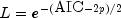, where p is the AR order, AIC is Akaike's Information Criterion, and L is the likelihood function evaluated for the optimum autoregressive model.
`Logger` `getLogger()`
Returns the logger object.
`int` `getMaxIterations()`
Returns the value currently being used as the maximum number of iterations allowed in the nonlinear equation solver used in both the method of moments and least-squares algorithms.
`int` `getMaxlag()`
Returns the current value used to represent the maximum number of autoregressive lags to achieve the minimum AIC.
`double` `getMean()`
Returns the mean used to center the time series `z`.
`int` `getOrder()`
Returns the order of the AR model selected with the minimum AIC.
`double[]` `getResiduals()`
Returns the current values of the vector of residuals.
`double[]` `getTimeSeries()`
Returns the time series used for estimating the minimum AIC and the autoregressive coefficients.
`double[]` `getTimsacAR()`
Returns the final auto regressive parameter estimates at the optimum AIC estimated by the original TIMSAC routine (UNIMAR).
`double` `getTimsacConstant()`
Returns the estimate for the constant parameter in the ARMA series.
`double` `getTimsacVariance()`
Returns the final estimate for the innovation variance calculated by the TIMSAC automatic AR modeling routine (UNIMAR).
`void` `setBackwardOrigin(int backwardOrigin)`
Sets the maximum backward origin used in calculating the forecasts.
`void` `setConfidence(double confidence)`
Sets the confidence level for calculating confidence limit deviations returned from `getDeviations`.
`void` `setConvergenceTolerance(double convergenceTolerance)`
Sets the tolerance level used to determine convergence of the nonlinear least-squares and maximum likelihood algorithms.
`void` `setEstimationMethod(int method)`
Sets the estimation method used for estimating the final estimates for the autoregressive coefficients.
`void` `setMaxIterations(int iterations)`
Sets the maximum number of iterations used for estimating the autoregressive coefficients.
`void` `setMean(double mean)`
Sets the estimate of the mean used for centering the time series ```z ```.
• ### Methods inherited from class java.lang.Object

`clone, equals, finalize, getClass, hashCode, notify, notifyAll, toString, wait, wait, wait`
• ### Field Detail

• #### LEAST_SQUARES

`public static final int LEAST_SQUARES`
Indicates that least-squares should be used for estimating the coefficients in the time series.
Constant Field Values
• #### MAX_LIKELIHOOD

`public static final int MAX_LIKELIHOOD`
Indicates that maximum likelihood should be used for estimating the coefficients in the time series.
Constant Field Values
• #### METHOD_OF_MOMENTS

`public static final int METHOD_OF_MOMENTS`
Indicates the method of moments should be used for estimating the coefficients in the time series.
Constant Field Values
• ### Constructor Detail

• #### ARAutoUnivariate

```public ARAutoUnivariate(int maxlag,
double[] z)```
`ARAutoUnivariate` constructor.
Parameters:
`maxlag` - an `int` scalar specifying the maximum number of autoregressive lags to evaluate
`z` - a `double` array containing the time series
• ### Method Detail

• #### compute

```public void compute()
throws ARMA.MatrixSingularException,
ARMA.TooManyCallsException,
ARMA.IncreaseErrRelException,
ARMA.NewInitialGuessException,
ARMA.IllConditionedException,
ARMA.TooManyITNException,
ARMA.TooManyFcnEvalException,
ARMA.TooManyJacobianEvalException,
ARAutoUnivariate.TriangularMatrixSingularException,
ARMAMaxLikelihood.NonStationaryException,
ARMAMaxLikelihood.NonInvertibleException,
ARMAMaxLikelihood.InitialMAException```
Determines the autoregressive model with the minimum AIC by fitting autoregressive models from 0 to `maxlag` lags using the method of moments or an estimation method specified by the user through ``` setEstimationMethod```.
Throws:
`ARMA.MatrixSingularException` - is thrown if the input matrix is singular.
`ARMA.TooManyCallsException` - is thrown if the number of calls to the function has exceeded the maximum number of iterations times the number of moving average (MA) parameters + 1.
`ARMA.IncreaseErrRelException` - is thrown if the bound for the relative error is too small.
`ARMA.NewInitialGuessException` - is thrown if the iteration has not made good progress.
`ARMA.IllConditionedException` - is thrown if the problem is ill-conditioned.
`ARMA.TooManyITNException` - is thrown if the maximum number of iterations is exceeded.
`ARMA.TooManyFcnEvalException` - is thrown if the maximum number of function evaluations is exceeded.
`ARMA.TooManyJacobianEvalException` - is thrown if the maximum number of Jacobian evaluations is exceeded.
`ARAutoUnivariate.TriangularMatrixSingularException` - is thrown if the input triangular matrix is singular.
`ARMAMaxLikelihood.NonStationaryException` - is thrown if the final maximum likelihood estimates for the time series are nonstationary
`ARMAMaxLikelihood.NonInvertibleException` - is thrown if the final maximum likelihood estimates for the time series are noninvertible.
`ARMAMaxLikelihood.InitialMAException` - is thrown if the initial values provided for the moving average terms using `setMA` are noninvertible. In this case, `ARMAMaxLikelihood` terminates and does not compute the time series estimates.
• #### forecast

```public double[][] forecast(int nForecast)
throws ARMA.MatrixSingularException,
ARMA.TooManyCallsException,
ARMA.IncreaseErrRelException,
ARMA.NewInitialGuessException,
ARMA.IllConditionedException,
ARMA.TooManyITNException,
ARMA.TooManyFcnEvalException,
ARMA.TooManyJacobianEvalException,
ARAutoUnivariate.TriangularMatrixSingularException,
ARMAMaxLikelihood.NonStationaryException,
ARMAMaxLikelihood.NonInvertibleException,
ARMAMaxLikelihood.InitialMAException```
Returns forecasts and associated confidence interval offsets.
Parameters:
`nForecast` - an input `int` representing the number of requested forecasts
Returns:
a `double` matrix of dimension `nForecast` by `backwardOrigin + 1` containing the forecasts. The forecasts are for lead times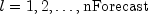at origins ``` z.length-backwardOrigin-1+j``` where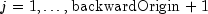.
Throws:
`ARMA.MatrixSingularException` - is thrown if the input matrix is singular
`ARMA.TooManyCallsException` - is thrown if the number of calls to the function has exceeded the maximum number of iterations times the number of moving average (MA) parameters + 1
`ARMA.IncreaseErrRelException` - is thrown if the bound for the relative error is too small
`ARMA.NewInitialGuessException` - is thrown if the iteration has not made good progress
`ARMA.IllConditionedException` - is thrown if the problem is ill-conditioned
`ARMA.TooManyITNException` - is thrown if the maximum number of iterations is exceeded
`ARMA.TooManyFcnEvalException` - is thrown if the maximum number of function evaluations is exceeded
`ARMA.TooManyJacobianEvalException` - is thrown if the maximum number of Jacobian evaluations is exceeded
`ARAutoUnivariate.TriangularMatrixSingularException` - is thrown if the input triangular matrix is singular
`ARMAMaxLikelihood.NonStationaryException` - is thrown if the final maximum likelihood estimates for the time series are nonstationary
`ARMAMaxLikelihood.NonInvertibleException` - is thrown if the final maximum likelihood estimates for the time series are noninvertible
`ARMAMaxLikelihood.InitialMAException` - is thrown if the initial values provided for the moving average terms using ``` setMA``` are noninvertible. In this case, ``` ARMAMaxLikelihood``` terminates and does not compute the time series estimates.
• #### getAIC

```public double getAIC()
throws ARMA.MatrixSingularException,
ARMA.TooManyCallsException,
ARMA.IncreaseErrRelException,
ARMA.NewInitialGuessException,
ARMA.IllConditionedException,
ARMA.TooManyITNException,
ARMA.TooManyFcnEvalException,
ARMA.TooManyJacobianEvalException,
ARAutoUnivariate.TriangularMatrixSingularException,
ARMAMaxLikelihood.NonStationaryException,
ARMAMaxLikelihood.NonInvertibleException,
ARMAMaxLikelihood.InitialMAException```
Returns the final estimate for Akaike's Information Criterion (AIC) at the optimum.
Returns:
a `double` scalar value which is an approximation to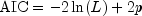, where L is the value of the maximum likelihood function evaluated at the parameter estimates. The approximation uses the calculation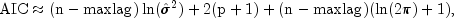whereis an estimate of the residual variance of the series, commonly known in time series analysis as the innovation variance, and `n` is the number of observations in the time series (``` n=z.length```).
Throws:
`ARMA.MatrixSingularException` - is thrown if the input matrix is singular
`ARMA.TooManyCallsException` - is thrown if the number of calls to the function has exceeded the maximum number of iterations times the number of moving average (MA) parameters + 1
`ARMA.IncreaseErrRelException` - is thrown if the bound for the relative error is too small
`ARMA.NewInitialGuessException` - is thrown if the iteration has not made good progress
`ARMA.IllConditionedException` - is thrown if the problem is ill-conditioned
`ARMA.TooManyITNException` - is thrown if the maximum number of iterations is exceeded
`ARMA.TooManyFcnEvalException` - is thrown if the maximum number of function evaluations is exceeded
`ARMA.TooManyJacobianEvalException` - is thrown if the maximum number of Jacobian evaluations is exceeded
`ARAutoUnivariate.TriangularMatrixSingularException` - is thrown if the input triangular matrix is singular
`ARMAMaxLikelihood.NonStationaryException` - is thrown if the final maximum likelihood estimates for the time series are nonstationary
`ARMAMaxLikelihood.NonInvertibleException` - is thrown if the final maximum likelihood estimates for the time series are noninvertible
`ARMAMaxLikelihood.InitialMAException` - is thrown if the initial values provided for the moving average terms using `setMA` are noninvertible. In this case, `ARMAMaxLikelihood` terminates and does not compute the time series estimates.
• #### getAR

```public double[] getAR()
throws ARMA.MatrixSingularException,
ARMA.TooManyCallsException,
ARMA.IncreaseErrRelException,
ARMA.NewInitialGuessException,
ARMA.IllConditionedException,
ARMA.TooManyITNException,
ARMA.TooManyFcnEvalException,
ARMA.TooManyJacobianEvalException,
ARAutoUnivariate.TriangularMatrixSingularException,
ARMAMaxLikelihood.NonStationaryException,
ARMAMaxLikelihood.NonInvertibleException,
ARMAMaxLikelihood.InitialMAException```
Returns the final auto regressive parameter estimates at the optimum AIC using the estimation method specified in ```setEstimationMethod ```.
Returns:
a `double` array containing the estimates for the autoregressive parameters.
Throws:
`ARMA.MatrixSingularException` - is thrown if the input matrix is singular.
`ARMA.TooManyCallsException` - is thrown if the number of calls to the function has exceeded the maximum number of iterations times the number of moving average (MA) parameters + 1.
`ARMA.IncreaseErrRelException` - is thrown if the bound for the relative error is too small.
`ARMA.NewInitialGuessException` - is thrown if the iteration has not made good progress.
`ARMA.IllConditionedException` - is thrown if the problem is ill-conditioned.
`ARMA.TooManyITNException` - is thrown if the maximum number of iterations is exceeded.
`ARMA.TooManyFcnEvalException` - is thrown if the maximum number of function evaluations is exceeded.
`ARMA.TooManyJacobianEvalException` - is thrown if the maximum number of Jacobian evaluations is exceeded.
`ARAutoUnivariate.TriangularMatrixSingularException` - is thrown if the input triangular matrix is singular.
`ARMAMaxLikelihood.NonStationaryException` - is thrown if the final maximum likelihood estimates for the time series are nonstationary.
`ARMAMaxLikelihood.NonInvertibleException` - is thrown if the final maximum likelihood estimates for the time series are noninvertible.
`ARMAMaxLikelihood.InitialMAException` - is thrown if the initial values provided for the moving average terms using ``` setMA``` are noninvertible. In this case, ``` ARMAMaxLikelihood``` terminates and does not compute the time series estimates.
• #### getBackwardOrigin

`public int getBackwardOrigin()`
Returns the maximum backward origin.
Returns:
an `int` scalar specifying the maximum backward origin.
• #### getConfidence

`public double getConfidence()`
Returns the confidence level for calculating confidence limit deviations returned from `getDeviations`.
Returns:
a `double` scalar value representing the confidence level used in computing forecast confidence intervals.
• #### getConstant

```public double getConstant()
throws ARMA.MatrixSingularException,
ARMA.TooManyCallsException,
ARMA.IncreaseErrRelException,
ARMA.NewInitialGuessException,
ARMA.IllConditionedException,
ARMA.TooManyITNException,
ARMA.TooManyFcnEvalException,
ARMA.TooManyJacobianEvalException,
ARAutoUnivariate.TriangularMatrixSingularException,
ARMAMaxLikelihood.NonStationaryException,
ARMAMaxLikelihood.NonInvertibleException,
ARMAMaxLikelihood.InitialMAException```
Returns the estimate for the constant parameter in the ARMA series.
Returns:
a `double` scalar equal to the estimate for the constant parameter in the ARMA series.
Throws:
`ARMA.MatrixSingularException` - is thrown if the input matrix is singular.
`ARMA.TooManyCallsException` - is thrown if the number of calls to the function has exceeded the maximum number of iterations times the number of moving average (MA) parameters + 1.
`ARMA.IncreaseErrRelException` - is thrown if the bound for the relative error is too small.
`ARMA.NewInitialGuessException` - is thrown if the iteration has not made good progress.
`ARMA.IllConditionedException` - is thrown if the problem is ill-conditioned.
`ARMA.TooManyITNException` - is thrown if the maximum number of iterations is exceeded.
`ARMA.TooManyFcnEvalException` - is thrown if the maximum number of function evaluations is exceeded.
`ARMA.TooManyJacobianEvalException` - is thrown if the maximum number of Jacobian evaluations is exceeded.
`ARAutoUnivariate.TriangularMatrixSingularException` - is thrown if the input triangular matrix is singular.
`ARMAMaxLikelihood.NonStationaryException` - is thrown if the final maximum likelihood estimates for the time series are nonstationary.
`ARMAMaxLikelihood.NonInvertibleException` - is thrown if the final maximum likelihood estimates for the time series are noninvertible.
`ARMAMaxLikelihood.InitialMAException` - is thrown if the initial values provided for the moving average terms using ``` setMA``` are noninvertible. In this case, ``` ARMAMaxLikelihood``` terminates and does not compute the time series estimates.
• #### getConvergenceTolerance

`public double getConvergenceTolerance()`
Returns the tolerance level used to determine convergence of the nonlinearleast-squares and maximum likelihood algorithms.
Returns:
a `double` scalar containing the tolerance level used to determine convergence of the nonlinear least-squares algorithm. `convergenceTolerance` represents the minimum relative decrease in sum of squares between two iterations required to determine convergence.
• #### getDeviations

`public double[] getDeviations()`
Returns the deviations used for calculating the forecast confidence limits.
Returns:
a `double` array of length ``` backwardOrigin+nForecast``` containing the deviations for calculating forecast confidence intervals. The confidence level is specified in `confidence`. By default, ``` confidence``` = 0.95.
• #### getEstimationMethod

`public int getEstimationMethod()`
Returns the estimation method used for estimating the autoregressive coefficients.
Returns:
an `int` equal to 0, 1, or 2, representing the autoregressive coefficient estimation method, which implies `METHOD_OF_MOMENTS`, `LEAST_SQUARES`, or `MAX_LIKELIHOOD` respectively.
• #### getForecast

```public double[] getForecast(int nForecast)
throws ARMA.MatrixSingularException,
ARMA.TooManyCallsException,
ARMA.IncreaseErrRelException,
ARMA.NewInitialGuessException,
ARMA.IllConditionedException,
ARMA.TooManyITNException,
ARMA.TooManyFcnEvalException,
ARMA.TooManyJacobianEvalException,
ARAutoUnivariate.TriangularMatrixSingularException,
ARMAMaxLikelihood.NonStationaryException,
ARMAMaxLikelihood.NonInvertibleException,
ARMAMaxLikelihood.InitialMAException```
Returns forecasts
Parameters:
`nForecast` - an input `int` representing the number of requested forecasts beyond the last value in the series.
Returns:
a `double` array containing the ``` nForecast+backwardOrigin``` forecasts. The first ``` backwardOrigin``` forecasts are one-step ahead forecasts for the last `backwardOrigin` values in the series. The next `nForecast` values in the returned series are forecasts for the next values beyond the series.
Throws:
`NonStationary` - is thrown if the final maximum likelihood estimates for the time series are nonstationary.
`NonInvertible` - is thrown if the final maximum likelihood estimates for the time series are noninvertible.
`InitialMAException` - is thrown if the initial values provided for the moving average terms using `setMA` are noninvertible. In this case, ```ARMAMaxLikelihood ``` terminates and does not compute the time series estimates.
`ARMA.MatrixSingularException` - is thrown if the input matrix is singular.
`ARMA.TooManyCallsException` - is thrown if the number of calls to the function has exceeded the maximum number of iterations times the number of moving average (MA) parameters + 1.
`ARMA.IncreaseErrRelException` - is thrown if the bound for the relative error is too small.
`ARMA.NewInitialGuessException` - is thrown if the iteration has not made good progress.
`ARMA.IllConditionedException` - is thrown if the problem is ill-conditioned.
`ARMA.TooManyITNException` - is thrown if the maximum number of iterations is exceeded.
`ARMA.TooManyFcnEvalException` - is thrown if the maximum number of function evaluations is exceeded.
`ARMA.TooManyJacobianEvalException` - is thrown if the maximum number of Jacobian evaluations is exceeded.
`ARAutoUnivariate.TriangularMatrixSingularException` - is thrown if the input triangular matrix is singular.
`ARMAMaxLikelihood.NonStationaryException` - is thrown if the final maximum likelihood estimates for the time series are nonstationary.
`ARMAMaxLikelihood.NonInvertibleException` - is thrown if the final maximum likelihood estimates for the time series are noninvertible.
`ARMAMaxLikelihood.InitialMAException` - is thrown if the initial values provided for the moving average terms using ``` setMA``` are noninvertible. In this case, ``` ARMAMaxLikelihood``` terminates and does not compute the time series estimates.
• #### getInnovationVariance

```public double getInnovationVariance()
throws ARMA.MatrixSingularException,
ARMA.TooManyCallsException,
ARMA.IncreaseErrRelException,
ARMA.NewInitialGuessException,
ARMA.IllConditionedException,
ARMA.TooManyITNException,
ARMA.TooManyFcnEvalException,
ARMA.TooManyJacobianEvalException,
ARAutoUnivariate.TriangularMatrixSingularException,
ARMAMaxLikelihood.NonStationaryException,
ARMAMaxLikelihood.NonInvertibleException,
ARMAMaxLikelihood.InitialMAException```
Returns the final estimate for the innovation variance.
Returns:
a `double` scalar value equal to the estimate for the innovation variance.
Throws:
`ARMA.MatrixSingularException` - is thrown if the input matrix is singular.
`ARMA.TooManyCallsException` - is thrown if the number of calls to the function has exceeded the maximum number of iterations times the number of moving average (MA) parameters + 1.
`ARMA.IncreaseErrRelException` - is thrown if the bound for the relative error is too small.
`ARMA.NewInitialGuessException` - is thrown if the iteration has not made good progress.
`ARMA.IllConditionedException` - is thrown if the problem is ill-conditioned.
`ARMA.TooManyITNException` - is thrown if the maximum number of iterations is exceeded.
`ARMA.TooManyFcnEvalException` - is thrown if the maximum number of function evaluations is exceeded.
`ARMA.TooManyJacobianEvalException` - is thrown if the maximum number of Jacobian evaluations is exceeded.
`ARAutoUnivariate.TriangularMatrixSingularException` - is thrown if the input triangular matrix is singular.
`ARMAMaxLikelihood.NonStationaryException` - is thrown if the final maximum likelihood estimates for the time series are nonstationary.
`ARMAMaxLikelihood.NonInvertibleException` - is thrown if the final maximum likelihood estimates for the time series are noninvertible.
`ARMAMaxLikelihood.InitialMAException` - is thrown if the initial values provided for the moving average terms using ``` setMA``` are noninvertible. In this case, ``` ARMAMaxLikelihood``` terminates and does not compute the time series estimates.
• #### getLikelihood

```public double getLikelihood()
throws ARMA.MatrixSingularException,
ARMA.TooManyCallsException,
ARMA.IncreaseErrRelException,
ARMA.NewInitialGuessException,
ARMA.IllConditionedException,
ARMA.TooManyITNException,
ARMA.TooManyFcnEvalException,
ARMA.TooManyJacobianEvalException,
ARAutoUnivariate.TriangularMatrixSingularException,
ARMAMaxLikelihood.NonStationaryException,
ARMAMaxLikelihood.NonInvertibleException,
ARMAMaxLikelihood.InitialMAException```
Returns the final estimate for, where p is the AR order, AIC is Akaike's Information Criterion, and L is the likelihood function evaluated for the optimum autoregressive model. If `MAX_LIKELIHOOD` is set in the `setEstimationMethod`, the exact likelihood evaluated for the optimum autoregressive model will be returned. Otherwise it is calculated using the approximation to the AIC.
Returns:
a `double` scalar equal to the maximum of the likelihood function,.
Throws:
`ARMA.MatrixSingularException` - is thrown if the input matrix is singular.
`ARMA.TooManyCallsException` - is thrown if the number of calls to the function has exceeded the maximum number of iterations times the number of moving average (MA) parameters + 1.
`ARMA.IncreaseErrRelException` - is thrown if the bound for the relative error is too small.
`ARMA.NewInitialGuessException` - is thrown if the iteration has not made good progress.
`ARMA.IllConditionedException` - is thrown if the problem is ill-conditioned.
`ARMA.TooManyITNException` - is thrown if the maximum number of iterations is exceeded.
`ARMA.TooManyFcnEvalException` - is thrown if the maximum number of function evaluations is exceeded.
`ARMA.TooManyJacobianEvalException` - is thrown if the maximum number of Jacobian evaluations is exceeded.
`ARAutoUnivariate.TriangularMatrixSingularException` - is thrown if the input triangular matrix is singular.
`ARMAMaxLikelihood.NonStationaryException` - is thrown if the final maximum likelihood estimates for the time series are nonstationary.
`ARMAMaxLikelihood.NonInvertibleException` - is thrown if the final maximum likelihood estimates for the time series are noninvertible.
`ARMAMaxLikelihood.InitialMAException` - is thrown if the initial values provided for the moving average terms using ``` setMA``` are noninvertible. In this case, ``` ARMAMaxLikelihood``` terminates and does not compute the time series estimates.
• #### getLogger

`public Logger getLogger()`
Returns the logger object.
Returns:
the logger object, if present, or null.
• #### getMaxIterations

`public int getMaxIterations()`
Returns the value currently being used as the maximum number of iterations allowed in the nonlinear equation solver used in both the method of moments and least-squares algorithms.
Returns:
an `int` containing the maximum number of iterations allowed.
• #### getMaxlag

`public int getMaxlag()`
Returns the current value used to represent the maximum number of autoregressive lags to achieve the minimum AIC.
Returns:
an `int` containing the maximum number of autoregressive lags evaluated.
• #### getMean

```public double getMean()
throws ARMA.MatrixSingularException,
ARMA.TooManyCallsException,
ARMA.IncreaseErrRelException,
ARMA.NewInitialGuessException,
ARMA.IllConditionedException,
ARMA.TooManyITNException,
ARMA.TooManyFcnEvalException,
ARMA.TooManyJacobianEvalException,
ARAutoUnivariate.TriangularMatrixSingularException,
ARMAMaxLikelihood.NonStationaryException,
ARMAMaxLikelihood.NonInvertibleException,
ARMAMaxLikelihood.InitialMAException```
Returns the mean used to center the time series `z`.
Returns:
a `double` containing the value used to center the series before searching for the optimum number of AR lags. This is equal to the mean of the time series `z` unless the mean was set in the `setMean` method.
Throws:
`ARMA.MatrixSingularException` - is thrown if the input matrix is singular.
`ARMA.TooManyCallsException` - is thrown if the number of calls to the function has exceeded the maximum number of iterations times the number of moving average (MA) parameters + 1.
`ARMA.IncreaseErrRelException` - is thrown if the bound for the relative error is too small.
`ARMA.NewInitialGuessException` - is thrown if the iteration has not made good progress.
`ARMA.IllConditionedException` - is thrown if the problem is ill-conditioned.
`ARMA.TooManyITNException` - is thrown if the maximum number of iterations is exceeded.
`ARMA.TooManyFcnEvalException` - is thrown if the maximum number of function evaluations is exceeded.
`ARMA.TooManyJacobianEvalException` - is thrown if the maximum number of Jacobian evaluations is exceeded.
`ARAutoUnivariate.TriangularMatrixSingularException` - is thrown if the input triangular matrix is singular.
`ARMAMaxLikelihood.NonStationaryException` - is thrown if the final maximum likelihood estimates for the time series are nonstationary.
`ARMAMaxLikelihood.NonInvertibleException` - is thrown if the final maximum likelihood estimates for the time series are noninvertible.
`ARMAMaxLikelihood.InitialMAException` - is thrown if the initial values provided for the moving average terms using ``` setMA``` are noninvertible. In this case, ``` ARMAMaxLikelihood``` terminates and does not compute the time series estimates.
• #### getOrder

```public int getOrder()
throws ARMA.MatrixSingularException,
ARMA.TooManyCallsException,
ARMA.IncreaseErrRelException,
ARMA.NewInitialGuessException,
ARMA.IllConditionedException,
ARMA.TooManyITNException,
ARMA.TooManyFcnEvalException,
ARMA.TooManyJacobianEvalException,
ARAutoUnivariate.TriangularMatrixSingularException,
ARMAMaxLikelihood.NonStationaryException,
ARMAMaxLikelihood.NonInvertibleException,
ARMAMaxLikelihood.InitialMAException```
Returns the order of the AR model selected with the minimum AIC.
Returns:
an `int` containing the optimum AR order selected by `ARAutoUnivariate`'s minimum AIC selection.
Throws:
`ARMA.MatrixSingularException` - is thrown if the input matrix is singular.
`ARMA.TooManyCallsException` - is thrown if the number of calls to the function has exceeded the maximum number of iterations times the number of moving average (MA) parameters + 1.
`ARMA.IncreaseErrRelException` - is thrown if the bound for the relative error is too small.
`ARMA.NewInitialGuessException` - is thrown if the iteration has not made good progress.
`ARMA.IllConditionedException` - is thrown if the problem is ill-conditioned.
`ARMA.TooManyITNException` - is thrown if the maximum number of iterations is exceeded.
`ARMA.TooManyFcnEvalException` - is thrown if the maximum number of function evaluations is exceeded.
`ARMA.TooManyJacobianEvalException` - is thrown if the maximum number of Jacobian evaluations is exceeded.
`ARAutoUnivariate.TriangularMatrixSingularException` - is thrown if the input triangular matrix is singular.
`ARMAMaxLikelihood.NonStationaryException` - is thrown if the final maximum likelihood estimates for the time series are nonstationary.
`ARMAMaxLikelihood.NonInvertibleException` - is thrown if the final maximum likelihood estimates for the time series are noninvertible.
`ARMAMaxLikelihood.InitialMAException` - is thrown if the initial values provided for the moving average terms using ``` setMA``` are noninvertible. In this case, ``` ARMAMaxLikelihood``` terminates and does not compute the time series estimates.
• #### getResiduals

`public double[] getResiduals()`
Returns the current values of the vector of residuals.
Returns:
a `double` array of length ```backwardOrigin ``` containing the residuals for the last ``` backwardOrigin``` values in the time series. This array will be null unless `compute` and either the ``` forecast``` or `getForecast` methods are called previously.
• #### getTimeSeries

`public double[] getTimeSeries()`
Returns the time series used for estimating the minimum AIC and the autoregressive coefficients.
Returns:
a `double` array containing the time series values set in the constructor.
• #### getTimsacAR

```public double[] getTimsacAR()
throws ARMA.MatrixSingularException,
ARMA.TooManyCallsException,
ARMA.IncreaseErrRelException,
ARMA.NewInitialGuessException,
ARMA.IllConditionedException,
ARMA.TooManyITNException,
ARMA.TooManyFcnEvalException,
ARMA.TooManyJacobianEvalException,
ARAutoUnivariate.TriangularMatrixSingularException,
ARMAMaxLikelihood.NonStationaryException,
ARMAMaxLikelihood.NonInvertibleException,
ARMAMaxLikelihood.InitialMAException```
Returns the final auto regressive parameter estimates at the optimum AIC estimated by the original TIMSAC routine (UNIMAR). These estimates vary depending upon the value of `maxlag` since its value changes the length of the series used to estimate these parameters. Use the `getAR` method to obtain the best estimates from the estimation method specified by `setEstimationMethod` calculated using the maximum number of available observations.
Returns:
a `double` array of length p containing the estimates for the autoregressive parameters.
Throws:
`ARMA.MatrixSingularException` - is thrown if the input matrix is singular.
`ARMA.TooManyCallsException` - is thrown if the number of calls to the function has exceeded the maximum number of iterations times the number of moving average (MA) parameters + 1.
`ARMA.IncreaseErrRelException` - is thrown if the bound for the relative error is too small.
`ARMA.NewInitialGuessException` - is thrown if the iteration has not made good progress.
`ARMA.IllConditionedException` - is thrown if the problem is ill-conditioned.
`ARMA.TooManyITNException` - is thrown if the maximum number of iterations is exceeded.
`ARMA.TooManyFcnEvalException` - is thrown if the maximum number of function evaluations is exceeded.
`ARMA.TooManyJacobianEvalException` - is thrown if the maximum number of Jacobian evaluations is exceeded.
`ARAutoUnivariate.TriangularMatrixSingularException` - is thrown if the input triangular matrix is singular.
`ARMAMaxLikelihood.NonStationaryException` - is thrown if the final maximum likelihood estimates for the time series are nonstationary.
`ARMAMaxLikelihood.NonInvertibleException` - is thrown if the final maximum likelihood estimates for the time series are noninvertible.
`ARMAMaxLikelihood.InitialMAException` - is thrown if the initial values provided for the moving average terms using ``` setMA``` are noninvertible. In this case, ``` ARMAMaxLikelihood``` terminates and does not compute the time series estimates.
• #### getTimsacConstant

```public double getTimsacConstant()
throws ARMA.MatrixSingularException,
ARMA.TooManyCallsException,
ARMA.IncreaseErrRelException,
ARMA.NewInitialGuessException,
ARMA.IllConditionedException,
ARMA.TooManyITNException,
ARMA.TooManyFcnEvalException,
ARMA.TooManyJacobianEvalException,
ARAutoUnivariate.TriangularMatrixSingularException,
ARMAMaxLikelihood.NonStationaryException,
ARMAMaxLikelihood.NonInvertibleException,
ARMAMaxLikelihood.InitialMAException```
Returns the estimate for the constant parameter in the ARMA series.
Returns:
a `double` scalar equal to the estimate for the constant parameter in the ARMA series.
Throws:
`ARMA.MatrixSingularException` - is thrown if the input matrix is singular.
`ARMA.TooManyCallsException` - is thrown if the number of calls to the function has exceeded the maximum number of iterations times the number of moving average (MA) parameters + 1.
`ARMA.IncreaseErrRelException` - is thrown if the bound for the relative error is too small.
`ARMA.NewInitialGuessException` - is thrown if the iteration has not made good progress.
`ARMA.IllConditionedException` - is thrown if the problem is ill-conditioned.
`ARMA.TooManyITNException` - is thrown if the maximum number of iterations is exceeded.
`ARMA.TooManyFcnEvalException` - is thrown if the maximum number of function evaluations is exceeded.
`ARMA.TooManyJacobianEvalException` - is thrown if the maximum number of Jacobian evaluations is exceeded.
`ARAutoUnivariate.TriangularMatrixSingularException` - is thrown if the input triangular matrix is singular.
`ARMAMaxLikelihood.NonStationaryException` - is thrown if the final maximum likelihood estimates for the time series are nonstationary.
`ARMAMaxLikelihood.NonInvertibleException` - is thrown if the final maximum likelihood estimates for the time series are noninvertible.
`ARMAMaxLikelihood.InitialMAException` - is thrown if the initial values provided for the moving average terms using ``` setMA``` are noninvertible. In this case, ``` ARMAMaxLikelihood``` terminates and does not compute the time series estimates.
• #### getTimsacVariance

```public double getTimsacVariance()
throws ARMA.MatrixSingularException,
ARMA.TooManyCallsException,
ARMA.IncreaseErrRelException,
ARMA.NewInitialGuessException,
ARMA.IllConditionedException,
ARMA.TooManyITNException,
ARMA.TooManyFcnEvalException,
ARMA.TooManyJacobianEvalException,
ARAutoUnivariate.TriangularMatrixSingularException,
ARMAMaxLikelihood.NonStationaryException,
ARMAMaxLikelihood.NonInvertibleException,
ARMAMaxLikelihood.InitialMAException```
Returns the final estimate for the innovation variance calculated by the TIMSAC automatic AR modeling routine (UNIMAR). This variance depends upon the value of `maxlag` since the series length in this computation depends on its value. Use `getInnovationVariance` to return the innovation variance calculated using the maximum series length.
Returns:
a `double` scalar value equal to the estimate for the innovation variance.
Throws:
`ARMA.MatrixSingularException` - is thrown if the input matrix is singular.
`ARMA.TooManyCallsException` - is thrown if the number of calls to the function has exceeded the maximum number of iterations times the number of moving average (MA) parameters + 1.
`ARMA.IncreaseErrRelException` - is thrown if the bound for the relative error is too small.
`ARMA.NewInitialGuessException` - is thrown if the iteration has not made good progress.
`ARMA.IllConditionedException` - is thrown if the problem is ill-conditioned.
`ARMA.TooManyITNException` - is thrown if the maximum number of iterations is exceeded.
`ARMA.TooManyFcnEvalException` - is thrown if the maximum number of function evaluations is exceeded.
`ARMA.TooManyJacobianEvalException` - is thrown if the maximum number of Jacobian evaluations is exceeded.
`ARAutoUnivariate.TriangularMatrixSingularException` - is thrown if the input triangular matrix is singular.
`ARMAMaxLikelihood.NonStationaryException` - is thrown if the final maximum likelihood estimates for the time series are nonstationary.
`ARMAMaxLikelihood.NonInvertibleException` - is thrown if the final maximum likelihood estimates for the time series are noninvertible.
`ARMAMaxLikelihood.InitialMAException` - is thrown if the initial values provided for the moving average terms using ``` setMA``` are noninvertible. In this case, ``` ARMAMaxLikelihood``` terminates and does not compute the time series estimates.
• #### setBackwardOrigin

`public void setBackwardOrigin(int backwardOrigin)`
Sets the maximum backward origin used in calculating the forecasts.
Parameters:
`backwardOrigin` - an `int` scalar specifying the maximum backward origin. ```backwardOrigin ``` must be greater than or equal to 0 and less than or equal to `z.length - p`, where `p` is the optimum number of AR coeficients determined by ``` ARAutoUnivariate```. The forecasted values returned will be ```z.length - backwardOrigin ``` through `z.length`. Default: `backwardOrigin = 0`.
• #### setConfidence

`public void setConfidence(double confidence)`
Sets the confidence level for calculating confidence limit deviations returned from `getDeviations`.
Parameters:
`confidence` - a `double` scalar specifying the confidence level used in computing forecast confidence intervals. Typical choices for ``` confidence``` are 0.90, 0.95, and 0.99. ``` confidence``` must be greater than 0.0 and less than 1.0. Default: `confidence = 0.95`.
• #### setConvergenceTolerance

`public void setConvergenceTolerance(double convergenceTolerance)`
Sets the tolerance level used to determine convergence of the nonlinear least-squares and maximum likelihood algorithms.
Parameters:
`convergenceTolerance` - a `double` scalar containing the tolerance level used to determine convergence of the nonlinear least-squares and maximum likelihood algorithms. `convergenceTolerance` represents the minimum relative decrease in sum of squares between two iterations required to determine convergence. Hence, `convergenceTolerance` must be greater than or equal to 0. By default, convergenceTolerance =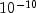.
• #### setEstimationMethod

`public void setEstimationMethod(int method)`
Sets the estimation method used for estimating the final estimates for the autoregressive coefficients.
Parameters:
`method` - an `int` scalar specifying the estimation method. Specify ``` METHOD_OF_MOMENTS``` for the method of moments, `LEAST_SQUARES` for the least squares method, or `MAX_LIKELIHOOD` for the maximum likelihood estimates. Default: `method` = `LEAST_SQUARES`.
• #### setMaxIterations

`public void setMaxIterations(int iterations)`
Sets the maximum number of iterations used for estimating the autoregressive coefficients.
Parameters:
`iterations` - an `int` scalar specifying the maximum number of iterations allowed in the nonlinear equation solver used in both the method of moments and least-squares algorithms. Default: ``` iterations``` = 300.
Throws:
`IllegalArgumentException` - is thrown if ``` iterations``` is less than or equal to 0.
• #### setMean

`public void setMean(double mean)`
Sets the estimate of the mean used for centering the time series ```z ```.
Parameters:
`mean` - a `double` containing the estimate of the mean for the time series `z`. By default, the time series `z` is centered about its sample mean.
JMSLTM Numerical Library 7.2.0